# HashMap死循环问题

package com.majing.java.concurrent;

import java.util.HashMap;
import java.util.Map;
import java.util.concurrent.atomic.AtomicInteger;

public class HashMapConcurrentProblem extends Thread{

private static Map<Integer, Integer> map = new HashMap<Integer, Integer>();
private static AtomicInteger at = new AtomicInteger(0);

@Override
public void run() {
while(at.get()<1000000){
map.put(at.get(), at.get());
at.incrementAndGet();
}
}

public static void main(String[] args) {
for(int i=0;i<10;i++){
Thread thread = new HashMapConcurrentProblem();
thread.start();
}
}

}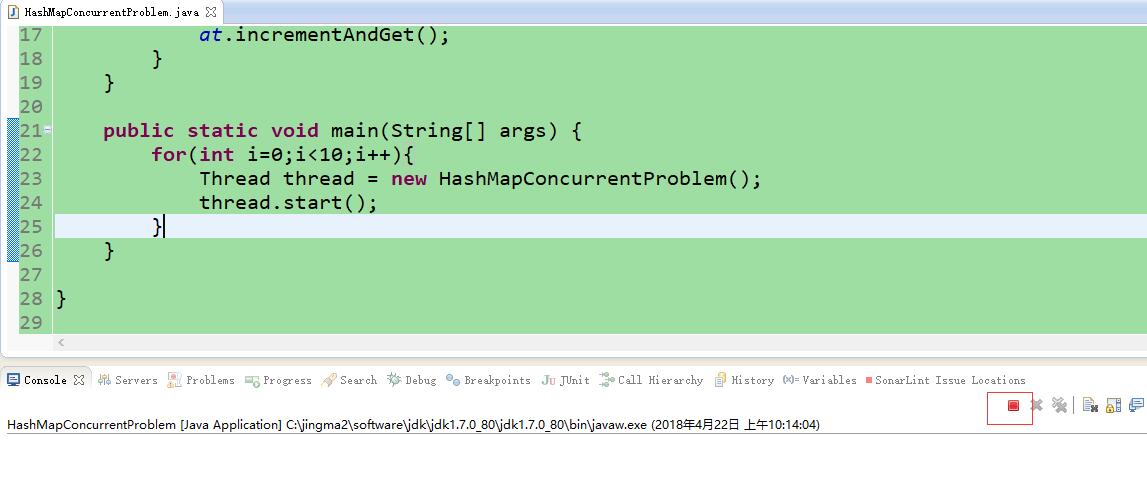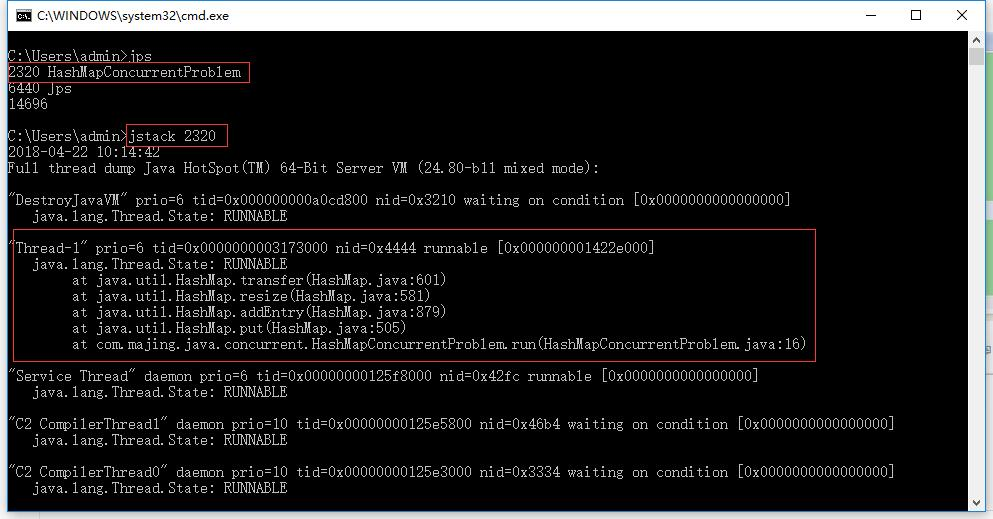1、假设我们的hash算法是简单的key mod一下表的大小（即数组的长度）。

2、最上面是old hash表，其中HASH表的size=2，所以key=3,5,7在mod 2 以后都冲突在table这个位置上了。

3、接下来HASH表扩容，resize=4，然后所有的<key,value>重新进行散列分布，过程如下：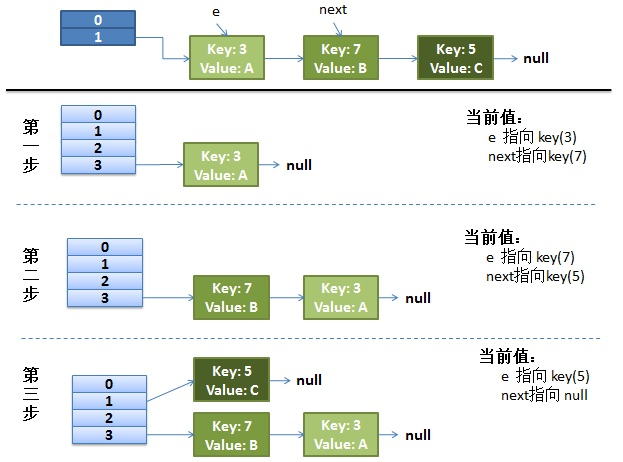void resize(int newCapacity) {
Entry[] oldTable = table;
int oldCapacity = oldTable.length;
if (oldCapacity == MAXIMUM_CAPACITY) {
threshold = Integer.MAX_VALUE;
return;
}

Entry[] newTable = new Entry[newCapacity];
transfer(newTable, initHashSeedAsNeeded(newCapacity));
table = newTable;
threshold = (int)Math.min(newCapacity * loadFactor, MAXIMUM_CAPACITY + 1);
}

/**
* Transfers all entries from current table to newTable.
*/
void transfer(Entry[] newTable, boolean rehash) {
int newCapacity = newTable.length;
for (Entry<K,V> e : table) {
while(null != e) {
Entry<K,V> next = e.next;
if (rehash) {
e.hash = null == e.key ? 0 : hash(e.key);
}
int i = indexFor(e.hash, newCapacity);
e.next = newTable[i];
newTable[i] = e;
e = next;
}
}
}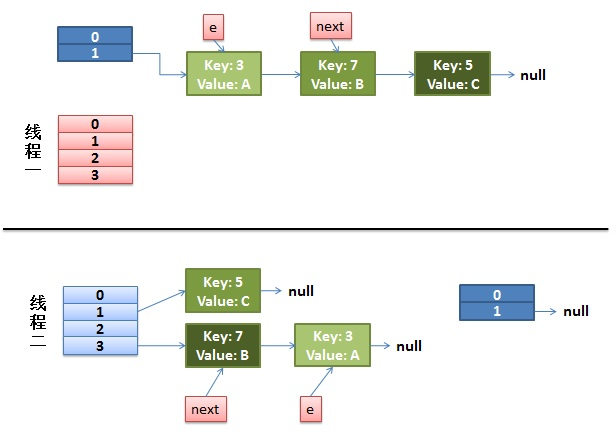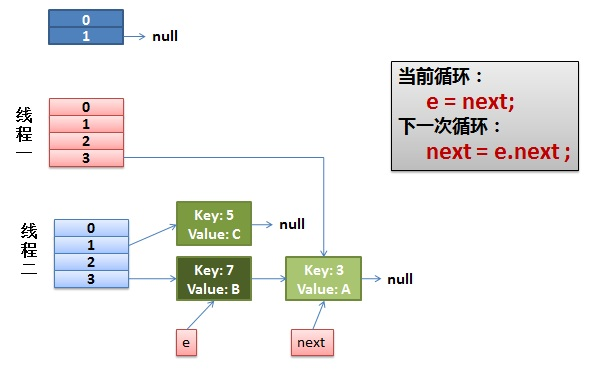newTable[i] = e;
e = next;

Entry<K,V> next = e.next;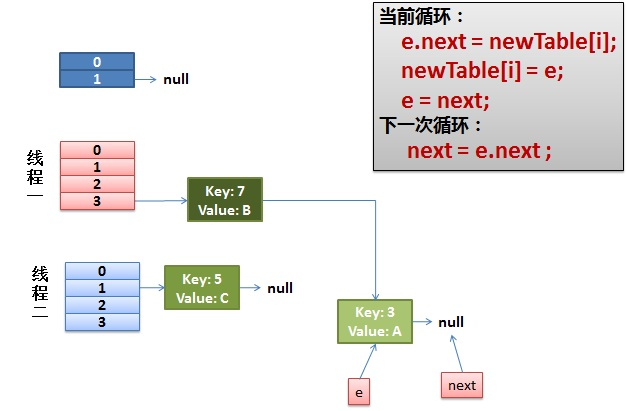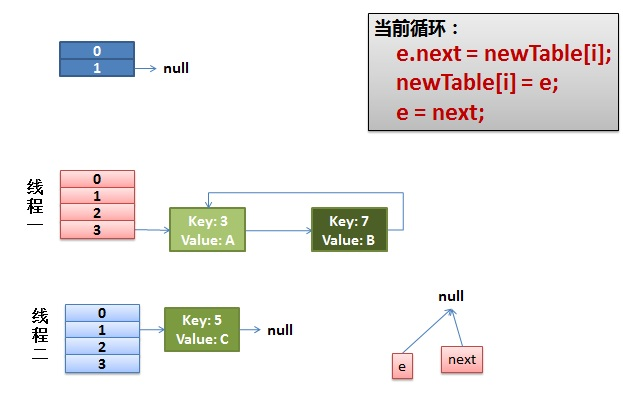09-142万+08-257130
08-091万+
01-23986
09-265621
03-0577
03-29158
11-052万+
07-275788
04-099902
©️2020 CSDN 皮肤主题: 程序猿惹谁了 设计师:白松林